# STARS

Every galaxy in the universe contains millions and millions of stars. A star is a ball of tremendous hot gas, which produces heat and light from nuclear reactions within its core. The closest star to the EARTH is the SUN.

# Here are examples of how to calculate orbital velocity of a given radius

## Orbital velocity in general relativity

The orbital velocity for a circular orbit with radius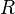is given by the following formula:whereis the Schwarzschild radius of the central body.

### Derivation

For the sake of convenience, the derivation will be written in units in which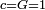.

### Time Derivation Notation:

A variety of notations are used to denote the time derivative.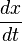A very common short-hand notation used, especially in mathematics and physics, is the ‘over-dot’. I.E.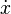(This is called Newton’s notation)

Higher time derivatives are also used: the second derivative with respect to time is written aswith the corresponding shorthand of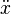.

As a generalization, the time derivative of a vector, say:is defined as the vector whose components are the derivatives of the components of the original vector. That is,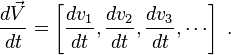The four-velocity of a body on a circular orbit is given by:(is constant on a circular orbit, and the coordinates can be chosen so that). The dot above a variable denotes derivation with respect to proper time.

For a massive particle, the components of the four-velocity satisfy the following equation:We use the geodesic equation: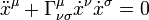The only nontrivial equation is the one for. It gives:From this, we get:Substituting this into the equation for a massive particle gives: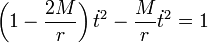Hence: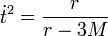Assume we have an observer at radius, who is not moving with respect to the central body, that is, his four-velocity is proportional to the vector. The normalization condition implies that it is equal to:The dot product of the four-velocities of the observer and the orbiting body equals the gamma factor for the orbiting body relative to the observer, hence:This gives the velocity: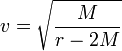Or, in SI units:## Circular acceleration

Transverse acceleration perpendicular to velocity causes change in direction. If it is constant in magnitude and changing in direction with the velocity, we get a circular motion. For this centripetal acceleration we havewhere:

•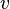is orbital velocity of orbiting body,
•is radius of the circle
•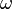is angular speed, measured in radians per unit time.

The formula is dimensionless, describing a ratio true for all units of measure applied uniformly across the formula. If the numerical value of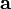is measured in meters per second per second, then the numerical values forwill be in meters per second,in meters, andin radians per second.

## Velocity

The relative velocity is constant: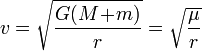where:

• G is the gravitational constant
• M and m are the masses of the orbiting bodies.
•is the standard gravitational parameter.

## Equation of motion

The orbit equation in polar coordinates, which in general gives r in terms of θ, reduces to:where:

•is specific angular momentum of the orbiting body.

This is just another way of writing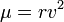again.

## Angular speed and orbital periodHence the orbital period(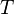) can be computed as:Compare two proportional quantities, the free-fall time (time to fall to a point mass from rest)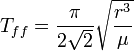(17.7 % of the orbital period in a circular orbit)

and the time to fall to a point mass in a radial parabolic orbit(7.5 % of the orbital period in a circular orbit)

The fact that the formulas only differ by a constant factor it is clear from dimensional analysis.

## Energy

The specific orbital energy () is negative, and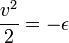Thus the virial theorem applies even without taking a time-average:

• the kinetic energy of the system is equal to the absolute value of the total energy
• the potential energy of the system is equal to twice the total energy

The escape velocity from any distance is √2 times the speed in a circular orbit at that distance: the kinetic energy is twice as much, hence the total energy is zero.

How to enter dot(s) or tilde notations above a time derivative – refer to Keyboards below this link.